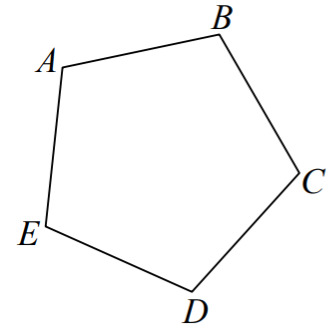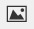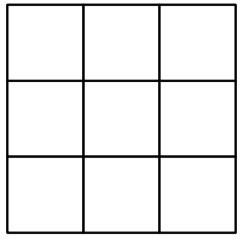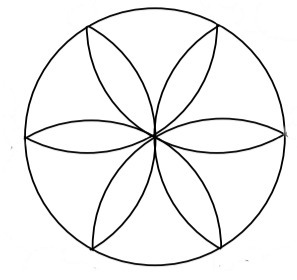PSA2PD2 - Problem Sets---Geometry, M1 Lesson 15 (G.CO.A.3)
Part A)

Use the figure below to answer the following questions.Draw all lines of symmetry. Locate the center of rotational symmetry.

Draw your answer on paper, take a picture, and upload it using the image upload icon:If you do not have the ability to upload an image of your work type "Answer is on paper."

Modified from EngageNY ©Great Minds Disclaimer

Part B)

Describe all symmetries explicitly.

What kinds are there?

Part C)

How many are rotations (including the identity symmetry)?

Type your answer below as a number (example: 5, 3.1, 4 1/2, or 3/2):
Part D)

How many are reflections?

Type your answer below as a number (example: 5, 3.1, 4 1/2, or 3/2):
Part E)

Now that you have found the symmetries of the pentagon, consider these questions:

How many places can vertex A be moved to by some symmetry of the pentagon? (Note that the vertex to which you move A by some specific symmetry is known as the image of A under that symmetry. Did you remember the identity symmetry?)

Type your answer below as a number (example: 5, 3.1, 4 1/2, or 3/2):
Part F)

For a given symmetry, if you know the image of A, how many possibilities exist for the image of B?

Part G)

Verify that there is symmetry for all possible images of A and B.

Part H)

Count the number of possible images of A and B. This is the total number of symmetries of the figure.

Type your answer below as a number (example: 5, 3.1, 4 1/2, or 3/2):
Part A)

Use the figure below to answer the following questions.Shade exactly two of the nine smaller squares so that the resulting figure has only one vertical and one horizontal line of symmetry.

Draw your answer on paper, take a picture, and upload it using the image upload icon:If you do not have the ability to upload an image of your work type "Answer is on paper."

Modified from EngageNY ©Great Minds Disclaimer

Part B)

Shade exactly two of the nine smaller squares so that the resulting figure has only two lines of symmetry about the diagonals.

Draw your answer on paper, take a picture, and upload it using the upload iconIf you do not have the ability to upload an image of your work, type "Answer is on paper."

Part C)

Shade exactly two of the nine smaller squares so that the resulting figure has only one horizontal line of symmetry.

Draw your answer on paper, take a picture, and upload it using the upload iconIf you do not have the ability to upload an image of your work, type "Answer is on paper."

Part D)

Shade exactly two of the nine smaller squares so that the resulting figure has only one line of symmetry about a diagonal.

Draw your answer on paper, take a picture, and upload it using the upload iconIf you do not have the ability to upload an image of your work, type "Answer is on paper."

Part E)

Shade exactly two of the nine smaller squares so that the resulting figure has no line of symmetry.

Draw your answer on paper, take a picture, and upload it using the upload iconIf you do not have the ability to upload an image of your work, type "Answer is on paper."

Part A)

Use the figure below to answer the following questions.Describe all the symmetries explicitly.

How many are rotations (including the identity symmetry)?

Modified from EngageNY ©Great Minds Disclaimer

Type your answer below as a number (example: 5, 3.1, 4 1/2, or 3/2):
Part B)

How many are reflections?

Type your answer below as a number (example: 5, 3.1, 4 1/2, or 3/2):
Part C)

How could you shade the figure so that the resulting figure only has three possible rotational symmetries (including the identity symmetry)?

Draw your answer on paper, take a picture, and upload it using the image upload icon:If you do not have the ability to upload an image of your work type "Answer is on paper."

Part A)

Decide whether each of the statements is true or false. Provide a counterexample below if the answer is false.

If a figure has exactly two lines of symmetry, it has exactly two rotational symmetries (including the identity symmetry).

Modified from EngageNY ©Great Minds Disclaimer

Select one:
Part B)

If a figure has at least three lines of symmetry, it has at least three rotational symmetries (including the identity symmetry).

Select one:
Part C)

If a figure has exactly two rotational symmetries (including the identity symmetry), it has exactly two lines of symmetry.

Select one:
Part D)

Provide a counterexample.

If you would like to upload an image as part of your answer, draw your counterexample on paper, take a picture, and upload it using the upload iconIf you do not have the ability to upload an image of your work, type "Counterexample is on paper."

If you would like to upload an image as part of your answer, draw your counterexample on paper, take a picture, and upload it using the upload icon